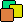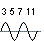Prime I.T. Help Prime I.T. :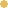Bitcoin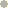Litecoin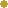DodgecoinVersion Française

The Sieve of Atkin

Note : This article has been translated using an automatic translator and one pass was made to remove biggest errors.
If you want to contribute to the readability of this article, you can notify me translations errors (a word, a sentence, a paragraph) by contacting me at the email address at the bottom of the page. Thanks

The principle

The Sieve of Atkin is a method considered as an optimized version of the Sieve of Eratosthenes to find all prime numbers up to a limit fixed N. The algorithm was recently developed by A. O. L. Atkin and Daniel J. Bernstein you can find the publication of their work "Prime sieves using binary quadratic forms" by following this link.

To understand the approach that the authors had on the design of the sieve, here is the "strategy" part of this document :
"...The idea of the new algorithm is to enumerate values of certain irreducible binary quadratic forms. For example, a squarefree positive integer p ∈ 1 + 4Z is prime if and only if the equation 4x² + y² = p has an odd number of positive solutions (x, y).
We cover all primes p > 3 as follows. For p ∈ 1 + 4Z we use 4x² + y² with x > 0 and y > 0, for p ∈ 7 + 12Z we use 3x² + y² with avec x > 0 and y > 0, for p ∈ 11 + 12Z we use 3x² - y² with avec x > y > 0...."

We see that the mathematical concepts that come into play are much more complex than Eratosthenes and unfortunately it is difficult to find relevant information on this sieve because very few popular article circulating on this algorithm. Moreover, this lack of information can also be found on Wikipedia, just go to the English version (Sieve of Atkin) and French (Crible d'Atkin) to see that the contents are not "synchronized" and for example the creation date of the sieve is different between the two texts (2004 vs 1999). May be due to a re-work?

First implementation

From a computational point of view, let's implement the version found in pseudo code in Wikipedia English in order to understand how it works. Despite the problem of content mentioned above, the site seems to be a good starting point for understanding Atkin.

private static final int MAX = 100000000;
private static final int SQRT_MAX = (intMath.sqrt(MAX1;
private static boolean[] array = new boolean[MAX];
//--//
for (int x = 1; x < SQRT_MAX; x++) {
for (int y = 1; y < SQRT_MAX; y++) {
int k = * x * x + y * y;
if ((k < MAX&& ((k % 12 == 1|| (k % 12 == 5))) {
array[k= !array[k];
}
k = * x * x + y * y;
if ((k < MAX&& (k % 12 == 7)) {
array[k= !array[k];
}
if (x > y) {
k = * x * x - y * y;
if ((k < MAX&& (k % 12 == 11)) {
array[k= !array[k];
}
}
}
}
array[2true;
array[3true;
for (int n = 5; n <= SQRT_MAX; n++) {
if (array[n]) {
int n2 = n * n;
for (int k = n2; k < MAX; k += n2) {
array[kfalse;
}
}
}

In terms of performance we have small hope because times of not optimized Atkin are better than Eratosthenes first implementation (and not optimized), we have 6 seconds 9 for Atkin against 7 seconds 5 for Eratosthenes (in the case of primes up to 100 million).
For cons the first optimization of Eratosthenes "hits hard" because the time of version 2 is divided by 2, can we do better with Atkin? (anxiety)

MAXPi(MAX)Time (sec)
1 000 00078 4980.063
10 000 000664 5790.625
100 000 0005 761 4556.953

Implementation, analysis time

To optimize Atkin, try to analyze the program and see where it takes more time. To do this we will first cut the first loop into 3 in order to calculate lap times. Of course the bursting of the loop does not influence the result, we will have all primes at the end of the program.
We also take the opportunity to redefine the upper bounds of 3 loops because the upper limit "square root" of the initial loop was a bit too wide and required the systematic addition of test "k < MAX". This first optimization does not save time but catching up the division into 3 loops.

double debut = System.currentTimeMillis();
int xUpper = (intMath.sqrt(MAX / 41;
for (int x = 1; x < xUpper; x++) {
for (int y = 1; y < Math.sqrt(MAX - k1); y++) {
Si (4x² + y²) % 12 == 1 ou (4x² + y²) % 12 == 5
--> Alors array[k] = !array[k];
}
}

double intermediaire1 = System.currentTimeMillis();
xUpper = (intMath.sqrt(MAX / 31;
for (int x = 1; x < xUpper; x++) {
for (int y = 2; y < Math.sqrt(MAX - k1); y++) {
Si (3x² + y²) % 12 == 7 Alors array[k] = !array[k];
}
}

double intermediaire2 = System.currentTimeMillis();
xUpper = (intMath.sqrt(MAX);
for (int x = 1; x < xUpper; x++) {
for (int y = 1; y < x; y++) {
Si (3x² - y²) % 12 == 11 Alors array[k] = !array[k];
}
}

double intermediaire3 = System.currentTimeMillis();
for (int n = 5; n <= SQRT_MAX; n++) {
Elimination des multiples des carrés n², 2n², 3n², ..., MAX
}
double fin = System.currentTimeMillis();

MAXPi(MAX)Time (sec) Loop 1 (sec)Loop 2 (sec)Loop 3 (sec)L. squares(sec)
1 000 00078 4980.062 0.0310.0150.00.016
10 000 000664 5790.656 0.2810.2340.0940.047
10 0000 0005 761 4556.984 3.0472.3281.0620.547

We see that the time of the loop 1 is greater than loop 2, itself greater than loop 3 and itself above the loop elimination of multiple squares. More loop 1 takes 43% of the total execution time of the algorithm, the loop 2 33%, the loop 3 15% and the loop of the squares 7%.
So we focus on the optimization of the first 3 loops because they are the biggest consumers and also because they resemble (in the sense optimization for one will certainly be applicable to others) and we will leave aside loop of the squares. source

Second implementation

To optimize the algorithm, we will address the modulo operator which is an expensive operation in computing time.
The idea is to be able to pass the test "modulo 12" present on the 3 loops and for that we will try to solve modular equations or at least find solutions in a form more simple for a computer.

What can we say about equations 4x² + y² = 1 / 5 , 3x² + y² = 7, 3x² - y² = 11 ?

• They have two unknowns x and y.
• They are modular.
• They are Diophantine equations, ie the coefficients are integers and solutions sought are also integers.
• They are non-linear (because of the degree 2).

Not knowing the mathematical tools to solve them, we "cheat" by pulling down the equations by hand to see what form are the solutions then try to formulate a rule. It is a kind of reverse engineering equations...

Equation 3x² + y² = 7 in the 2nde loop seems to be a good candidate to start. source

for (int x = 1; x < xUpper; x++) {
int k1 = * x * x;
for (int y = 2; y < Math.sqrt(MAX - k1); y++) {
int k = k1 + y * y;
if (k % 12 == 7) {
System.out.println("x = " + x + " y = " + y);
}
}
}
x = 55 y = 9988
x = 55 y = 9992
x = 55 y = 9994
x = 55 y = 9998
x = 57 y = 2
x = 57 y = 4
x = 57 y = 8
x = 57 y = 10
x = 57 y = 14

We see that the solutions meet the following rules :

• x must be odd.
• y takes the following values 2, 4, 8, 10, 14... ie que the difference between the solutions y seems to follow the sequence +2, +4, +2, +4....

Solutions being generalized, we can optimize the loop 2 :

int[] sequence2 = 4};

for (int x = 1; x < xUpper; x += 2) {
int index = 0;
int k1 = * x * x;
for (int y = 2; y < Math.sqrt(MAX - k1); y += sequence2[(++index & 1)]) {
int k = k1 + y * y;
array[k= !array[k];
}
}

And improvements are tangible. source

MAXLoop 2 (sec)
1 000 0000.0
10 000 0000.047
100 000 0000.5

The optimization gives good results as the loop is 5 times faster than the previous implementation but do not race this loop lent itself well to this change...

Third implementation

Now that we have seen how to improve the time loop 2, we can do the same on the other 2 loops.
We get the following rules :

For loop 1

• There are no specific rules for x.
• About y, equation admits "2 sets of solution" depending on whether x is divisible by 3 or not.
When x is divisible by 3, takes values 1, 5, 7, 11, 13... ie the gap seems to follow the sequence +4, +2, +4, +2....
When x is not divisible by 3, the solutions are odd.

For loop 3

• There are no specific rules for x.
• About y, equation admits "2 sets of solution" depending on whether x is even or not.
When x is even, the gap seems to follow solutions sequence +2, +4, +2, +4...
When x is odd, the gap seems to follow solutions sequence +4, +2, +4, +2....

Note the sequence [+2,+4,...] (and its equivalent [+4,+2,...]) present in all solutions y, we take the opportunity to pool this element in the optimized implementation.
And here are the results as expected...

MAXPi(MAX)Time (sec) Loop 1 (sec)Loop 2 (sec)Loop 3 (sec)L. squares(sec)
1 000 00078 4980.016 0.00.0160.00.0
10 000 000664 5790.187 0.0780.0310.0160.062
100 000 0005 761 4552.187 0.8590.3910.3440.593

Enjoy ! We managed to get a best time than Eratosthenes optimized. For comparison, the Eratosthenes "second implementation" is 3.063 seconds for a limit 100 million while Atkin is 2.187, we have a gain of just under 50%. source

Fourth implementation

Keep on running and trying to reach 1 billion by implementing Atkin with an array of bit, it is exactly the same approach that we had with the 3rd implementation of Eratosthenes. We will need 2 additional bit operations over Eratosthenes namely : unSetBit to unset a bit of the array and toggleBit to toogle a bit of the array. source

MAXPi(MAX)Time (sec) Loop 1 (sec)Loop 2 (sec)Loop 3 (sec)L. squares(sec)
1 000 00078 4970.016 0.00.00.00.016
10 000 000664 5780.172 0.0470.0150.0310.079
100 000 0005 761 4543.172 1.4220.5310.390.829
1 000 000 00050 847 53337.453 16.1097.1576.0158.172

For the moment it is still unclear but the complexity of the algorithm leads to 2 trails :

• Int vs long
To reach 1 billion some Java types are passed from int to long. Maybe this change is impacting more than expected and it appears in more complex calculations of Atkin ? (I think of x² or y²). Besides the times were measured on an old 32 bits machine.
• Too many calls to the handling bits.
Operations setBit, unSetBit, toggleBit are likely to be costly time, maybe Atkin "abuse" and often called ?

In conclusion

Nous voyons qu'atkin peut aller plus vite qu'eratosthène lorsqu'il est "savamment" optimisé mais que d'effort pour arriver à ce résultat. We see that atkin can go faster than Eratosthenes when it is "cleverly" optimized but so efforts to achieve this
The code is much less elegant than Eratosthenes, all that to save time.

A website created by Les composants associés (cpas)